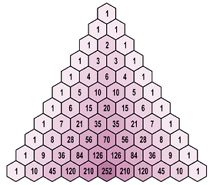Algebra

# Binomial Theorem: Level 4 Challenges

$\large \sum_{k=0}^n \left[ (-1)^k \dbinom{n}{k} (n-k)^n \right] = \ ?$$\large\displaystyle \sum_{k=1}^{504} \left ( 4k-1 \right ) \binom{2015}{4k-1}$

If the sum above can be written as $$p.q^{r}$$, where $$p$$, $$q$$ and $$r$$ are positive integers with $$q$$ being a prime.

Evaluate $$p+q+r$$.

###### Image Credit: Wikimedia TED-43.

Let a natural number $$n$$ be good if there exist two distinct non-integral real numbers $$a$$ and $$b$$ such that $$a^k - b^k$$ is an integer for all $$1 \leq k \leq n$$.

Find the number of natural numbers which are not good.

Compute the remainder when $\dbinom {2014}{1} + \dbinom {2014}{4} + \dbinom {2014}{7} + \cdots + \dbinom {2014}{2014}$ is divided by $$1000$$.

Find the number of odd coefficients in the expansion of $$(a + b)^{2015}.$$

×